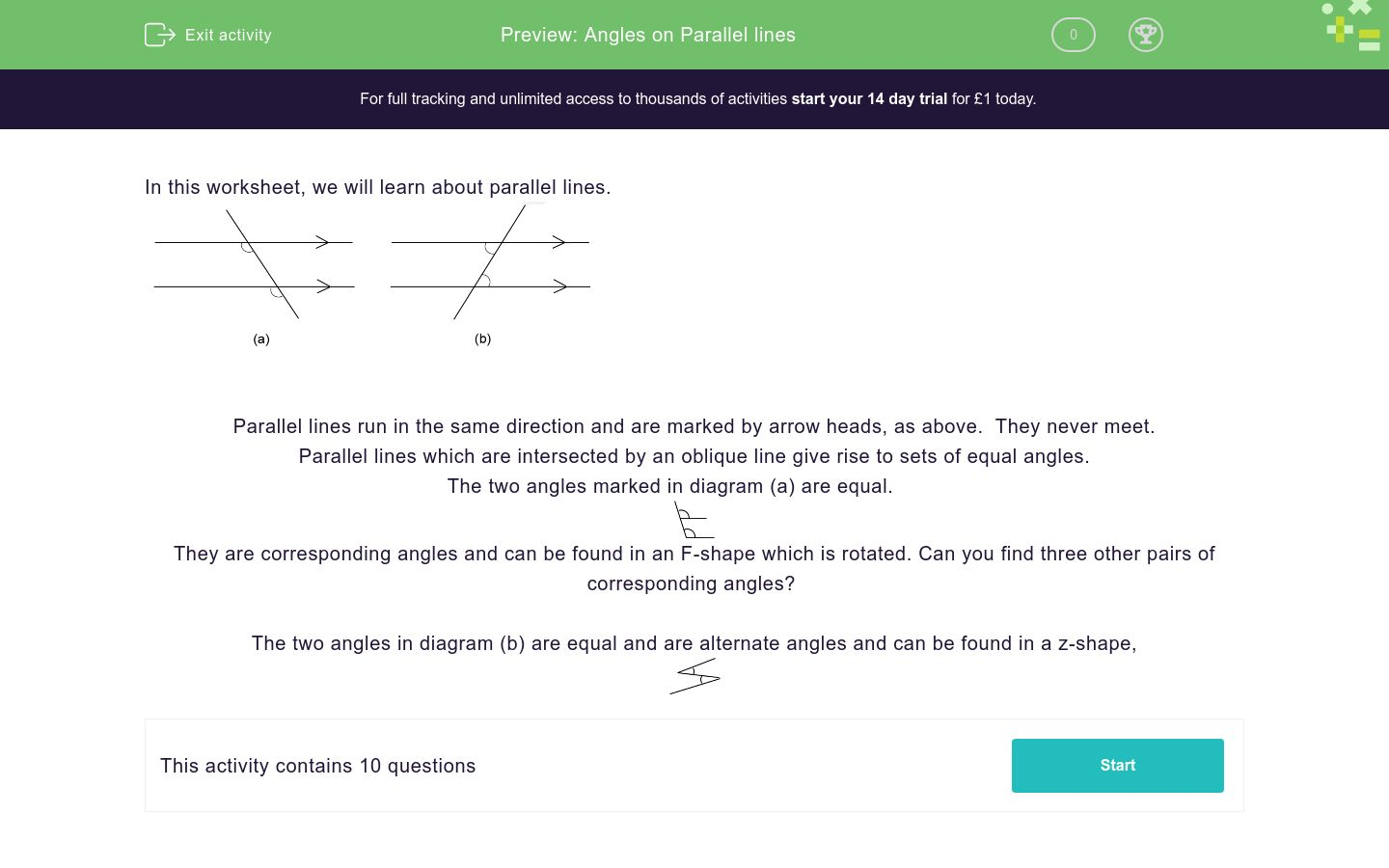# Angles on Parallel lines

In this worksheet, students answer questions on alternate and corresponding angles on parallel lines.Key stage:  KS 3

Curriculum topic:   Geometry and Measures

Curriculum subtopic:   Understand the Relationship Between Parallel Lines and Angles

Difficulty level:#### Worksheet Overview

In this worksheet, we will learn about parallel lines.Parallel lines run in the same direction and are marked by arrowheads, as above.  They never meet.

Parallel lines which are intersected by an oblique line give rise to sets of equal angles.

The two angles marked in diagram (a) are equal.They are corresponding angles and can be found in an F-shape which is rotated. Can you find three other pairs of corresponding angles?

The two angles in diagram (b) are equal and are alternate angles and can be found in a z-shape,### What is EdPlace?

We're your National Curriculum aligned online education content provider helping each child succeed in English, maths and science from year 1 to GCSE. With an EdPlace account you’ll be able to track and measure progress, helping each child achieve their best. We build confidence and attainment by personalising each child’s learning at a level that suits them.

Get started